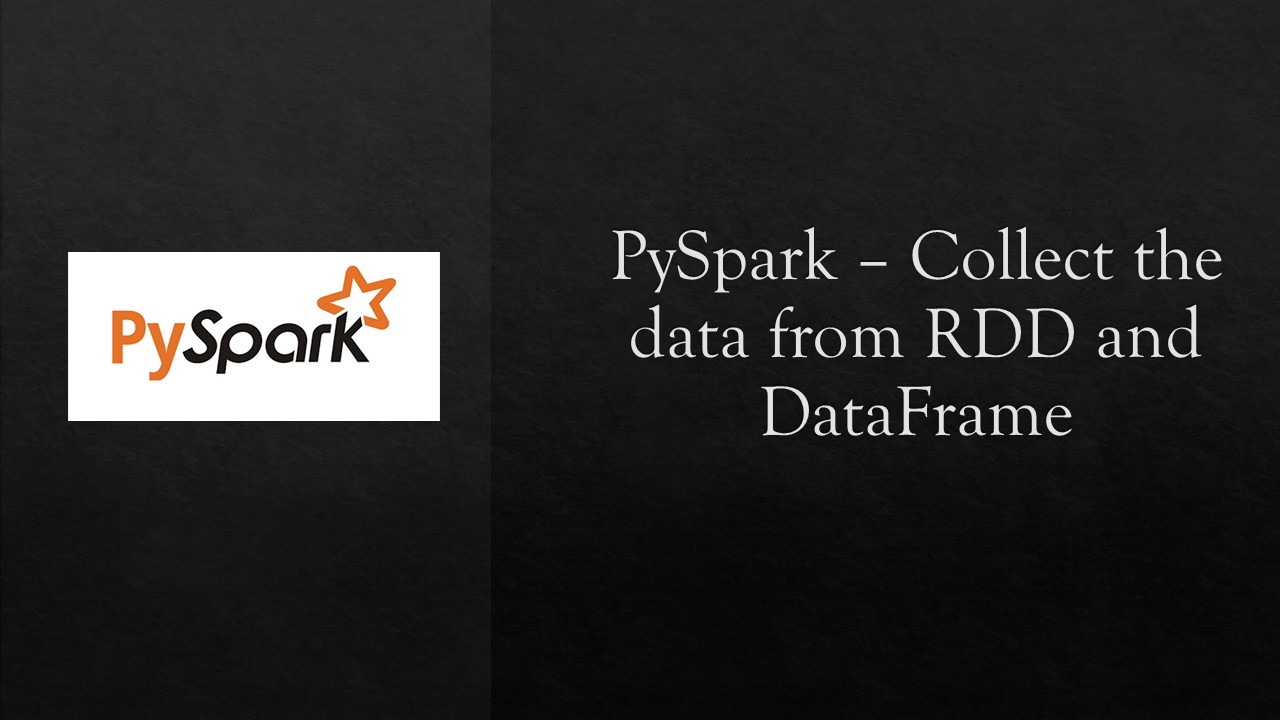# Collect() – Retrieve data from Spark RDD/DataFrame

Spark `collect()` and `collectAsList()` are action operation that is used to retrieve all the elements of the RDD/DataFrame/Dataset (from all nodes) to the driver node. We should use the collect() on smaller dataset usually after filter(), group(), count() e.t.c. Retrieving on larger dataset results in out of memory.

In this Spark article, I will explain the usage of `collect()` with DataFrame example, when to avoid it, and the difference between `collect()` and `select()`.

In order to explain with example, first, let’s create a DataFrame.

``````
val spark:SparkSession = SparkSession.builder()
.master("local")
.appName("SparkByExamples.com")
.getOrCreate()

val data = Seq(Row(Row("James ","","Smith"),"36636","M",3000),
Row(Row("Michael ","Rose",""),"40288","M",4000),
Row(Row("Robert ","","Williams"),"42114","M",4000),
Row(Row("Maria ","Anne","Jones"),"39192","F",4000),
Row(Row("Jen","Mary","Brown"),"","F",-1)
)

val schema = new StructType()

val df = spark.createDataFrame(spark.sparkContext.parallelize(data),schema)
df.printSchema()
df.show(false)
``````

`show()` function on DataFrame prints the result of the dataset in a table format. By default, it shows only 20 rows. The above snippet returns the data in a table.

``````
root
|-- name: struct (nullable = true)
|    |-- firstname: string (nullable = true)
|    |-- middlename: string (nullable = true)
|    |-- lastname: string (nullable = true)
|-- id: string (nullable = true)
|-- gender: string (nullable = true)
|-- salary: integer (nullable = true)

+---------------------+-----+------+------+
|name                 |id   |gender|salary|
+---------------------+-----+------+------+
|[James , , Smith]    |36636|M     |3000  |
|[Michael , Rose, ]   |40288|M     |4000  |
|[Robert , , Williams]|42114|M     |4000  |
|[Maria , Anne, Jones]|39192|F     |4000  |
|[Jen, Mary, Brown]   |     |F     |-1    |
+---------------------+-----+------+------+
``````

## Using collect() and collectAsList()

`collect()` action function is used to retrieve all elements from the dataset (RDD/DataFrame/Dataset) as a `Array[Row]` to the driver program.

`collectAsList()` action function is similar to collect() but it returns Java util list.

Syntax:

``````
collect() : scala.Array[T]
collectAsList() : java.util.List[T]
``````

#### collect() Example

``````
val colList = df.collectAsList()
val colData = df.collect()
colData.foreach(row=>
{
val salary = row.getInt(3)//Index starts from zero
println(salary)
})

``````

`deptDF.collect()` retrieves all elements in a DataFrame as an array to the driver. From the array, I’ve retried the firstName element and printed on the console.

``````
3000
4000
4000
4000
-1
``````

## Retrieving data from Struct column

To retrieve a struct column from `Row`, we should use `getStruct()` function.

``````
//Retrieving data from Struct column
colData.foreach(row=>
{
val salary = row.getInt(3)
val fullName:Row = row.getStruct(0) //Index starts from zero
val firstName = fullName.getString(0)//In struct row, again index starts from zero
val middleName = fullName.get(1).toString
val lastName = fullName.getAs[String]("lastname")
println(firstName+","+middleName+","+lastName+","+salary)
})
``````

Above example explains the use of different Row class functions `get()`, `getString()`, `getAs[String]()`, `getStruct()`.

``````
James ,,Smith,3000
Michael ,Rose,,4000
Robert ,,Williams,4000
Maria ,Anne,Jones,4000
Jen,Mary,Brown,-1
``````

Note that like other DataFrame functions, `collect()` does not return a Dataframe instead, it returns data in an array to your driver. once the data is collected in an array, you can use scala language for further processing.

In case you want to just return certain elements of a DataFrame, you should call select() first.

``````
dataCollect = df.select("name").collect()
``````

## When to avoid Collect()

Usually, collect() is used to retrieve the action output when you have very small result set and calling `collect()` on an RDD/DataFrame with a bigger result set causes out of memory as it returns the entire dataset (from all workers) to the driver hence we should avoid calling collect() on a larger dataset.

## collect () vs select ()

`select()` method on an RDD/DataFrame returns a new DataFrame that holds the columns that are selected whereas collect() returns the entire data set.

`select()` is a transformation function whereas `collect()` is an action.

## Complete Example of Spark collect()

Below is a complete Spark example of using `collect()` and `collectAsList()` on DataFrame, similarly, you can also create a program with RDD.

``````
import org.apache.spark.sql.{Row, SparkSession}
import org.apache.spark.sql.types.{IntegerType, StringType, StructType}

object CollectExample extends App {

val spark:SparkSession = SparkSession.builder()
.master("local")
.appName("SparkByExamples.com")
.getOrCreate()

val data = Seq(Row(Row("James ","","Smith"),"36636","M",3000),
Row(Row("Michael ","Rose",""),"40288","M",4000),
Row(Row("Robert ","","Williams"),"42114","M",4000),
Row(Row("Maria ","Anne","Jones"),"39192","F",4000),
Row(Row("Jen","Mary","Brown"),"","F",-1)
)

val schema = new StructType()

val df = spark.createDataFrame(spark.sparkContext.parallelize(data),schema)
df.printSchema()
df.show(false)

val colData = df.collect()

colData.foreach(row=>
{
val salary = row.getInt(3)//Index starts from zero
println(salary)
})

//Retrieving data from Struct column
colData.foreach(row=>
{
val salary = row.getInt(3)
val fullName:Row = row.getStruct(0) //Index starts from zero
val firstName = fullName.getString(0)//In struct row, again index starts from zero
val middleName = fullName.get(1).toString
val lastName = fullName.getAs[String]("lastname")
println(firstName+","+middleName+","+lastName+","+salary)
})
}
``````

This example is also available at Spark Github project.

## Conclusion

In this Spark article, you have learned the collect() and collectAsList() function of the RDD/DataFrame which returns all elements of the DataFrame to Driver program and also learned it’s not a good practice to use it on the bigger dataset, finally retrieved the data from Struct field.

Happy Learning !!

### NNK

SparkByExamples.com is a Big Data and Spark examples community page, all examples are simple and easy to understand and well tested in our development environment Read more ..

### This Post Has 4 Comments

1.Anonymous

Hi NNK, this site is the best one so far to learn spark. Thank You for the great effort.

2.Anonymous

If we cant use collect() for a large dataset, what we should use? thanks

1.NNK

Why would you want to collect a large dataset? When it doesn’t fit in memory you can’t even use it for anything else. Ideally, you should write the DataFrame to a File system, Kafka or to a database (Mongo, Casandra e.t.c)

3.Anonymous

If we cant use collect() for a large dataset, what we should use? thanks